# 含时滞的非线性脉冲切换系统的指数稳定性Exponential Stability of Impulsive Switched Nonlinear Systems with Time Delay: The Average Dwell Time Approach

DOI: 10.12677/ORF.2019.94038, PDF, HTML, XML, 下载: 245  浏览: 618

Abstract: This paper mainly tends to investigate the exponential stability problem for a class of impulsive switched systems with nonlinear perturbation and time delay which consist of both Hurwitz stable and unstable subsystems. Based on the average dwell time approach to study the exponential sta-bility of the system, The sufficient conditions of exponential stability are derived by using the given lemmas; some sufficient conditions are given to ensure that the state trajectory of the system remains in a bounded region over a prespecified finite time interval. A numerical example is em-ployed to show the efficiency of the theories.

1. 引言

2. 预备知识

R、 ${R}_{+}$${R}_{-}$ 分别代表全体实数、正实数、负实数。

${L}_{p}\left({R}_{+},{R}^{n}\right)=\left\{u|u:{R}_{+}\to {R}^{n},{|u|}_{p}={\left({\int }_{{R}_{+}}{‖u\left(t\right)‖}^{p}\text{d}t\right)}^{1/p}<+\infty \right\}$ , $1\le p<+\infty$ ,

${R}^{n×m}$ 代表所有 $n×m$ 实数矩阵，I为对应阶的单位矩阵。

$\begin{array}{l}\stackrel{˙}{x}\left(t\right)={A}_{\sigma \left(t\right)}x\left(t-\tau \right)+{f}_{\sigma \left(t\right)}\left(t,x\left(t\right)\right),\text{ }\text{ }\text{ }\text{ }\text{ }\text{ }\text{ }\text{ }\text{ }\text{ }\text{ }\text{ }\text{ }\text{ }\text{ }\text{ }\text{ }t\in \left({t}_{k-1},{t}_{k}\right],\text{ }\text{ }\text{ }\text{ }\text{ }\text{ }k=1,2,\cdots \\ \Delta x\left(t\right)=x\left({t}_{k}^{+}\right)-x\left({t}_{k}\right)={F}_{k}x\left(t\right),\text{ }\text{ }\text{ }\text{ }\text{ }\text{ }\text{ }\text{ }\text{ }\text{ }\text{ }\text{ }\text{ }\text{ }\text{ }\text{ }\text{ }\text{ }\text{ }\text{ }\text{ }\text{ }\text{ }\text{ }\text{ }\text{ }\text{ }\text{ }\text{ }\text{ }t={t}_{k}\\ x\left({t}_{0}^{+}\right)={x}_{0},\\ x\left(t\right)=h\left(t\right),\text{ }\text{ }\text{ }\text{ }\text{ }\text{ }\text{ }\text{ }t<0,\end{array}$ (1)

${|{f}_{i}\left(t,x\left(t\right)\right)|}_{2}\le m|x\left(t\right)|{\text{ }}_{2}$ , $i\in G$ ,

m是常数且 $m>0$${F}_{k}\left(k=1,2,\cdots \right)$ 是常数矩阵，代表在切换时刻所产生的脉冲结果，且 $0<‖{F}_{k}‖\le {\theta }_{k}$${N}_{\sigma }\left(\tau ,t\right)$ 表示切换信号 $\sigma$ 在区间 $\left(\tau ,t\right)$ 的切换次数，并且假设 $x\left({t}_{k}^{+}\right)={\mathrm{lim}}_{l\to {0}^{+}}x\left({t}_{k}+l\right)$

${N}_{\sigma }\left(u,t\right)\le {N}_{0}+\frac{t-u}{{\tau }_{a}}$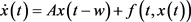, (2)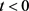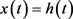;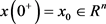, (3)

(i)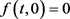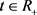(ii)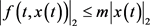, m是常数。,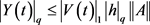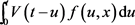满足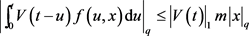,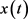取范数得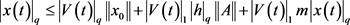, (4)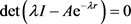, (5)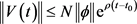，对于所有的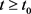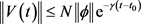，对于所有的3. 主要结论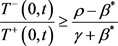, (6)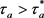，m满足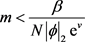，则系统(1)满足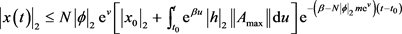,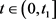利用引理1可得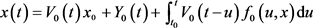,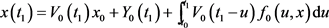.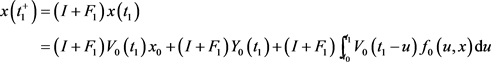.,...，利用引理2，可以得到.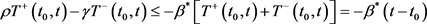..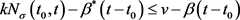,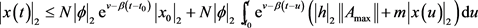,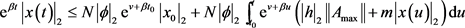,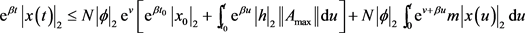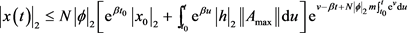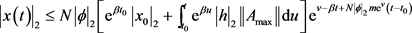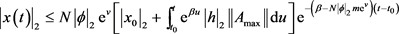4. 算例,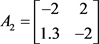,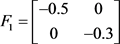,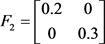,,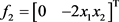,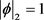，由不等式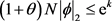可以求得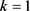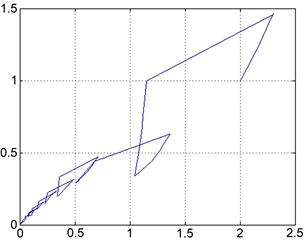Figure 1. The state trajectory of the systems that the average dwell time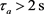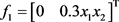,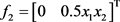,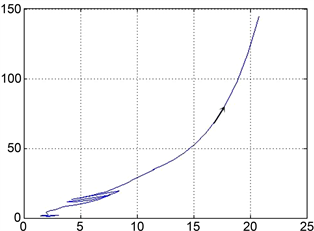Figure 2. The state trajectory of the systems that the average dwell time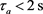5. 结论

  Zhu, L.Y. and Feng, G. (2015) Necessary and Sufficient Conditions for Stability of Switched Nonlinear Systems. Journal of the Franklin Institute, 352, 117-137. https://doi.org/10.1016/j.jfranklin.2014.10.011  Vu, L., Chatterjee, D. and Liberzon, D. (2007) Input-to-State Stability of Switched Systems and Switching Adaptive Control. Automatica, 43, 639-646. https://doi.org/10.1016/j.automatica.2006.10.007  Zhu, W. (2010) Stability Analysis of Switched Impulsive Systems with Time Delays. Nonlinear Analysis: Hybrid Systems, 4, 608-617. https://doi.org/10.1016/j.nahs.2010.03.009  Liberzon, D., Hespanha, J.P. and Morse, A.S. (1999) Stability of Switched Systems: A Lie-Algebraic Condition. Systems & Control Letters, 37, 117-122. https://doi.org/10.1016/S0167-6911(99)00012-2  Zhang, J.F., Han, Z.Z., Zhu, F.B. and Zhao, X.D. (2014) Absolute Exponential Stability and Stabilization of Switched Nonlinear Systems. Systems & Control Letters, 66, 51-57. https://doi.org/10.1016/j.sysconle.2013.12.021  秦元勋, 刘永清, 王联. 带有时滞的动力系统的稳定性[M]. 北京: 科学出版社, 1989.  Morse, A.S. (1996) Supervisory Control of Families of Linear Set-Point Controllers. IEEE Transactions on Automatic Control, 41, 1413-1431. https://doi.org/10.1109/9.539424  Hespanha, J.P. and Morse, A.S. (1999) Stability of Switched Systems with Average Dwell-Time. In: Proceedings of the 38th IEEE Conference on Decision and Control, IEEE, New York, 2655-2660.  Wang, Y.J., Shi, X.M. and Zuo, Z.Q. (2013) On Finite-Time Stability for Nonlinear Impulsive Switched Systems. Nonlinear Analysis, Real World Application, 14, 807-814. https://doi.org/10.1016/j.nonrwa.2012.08.003  赵世欣. 基于平均停留时间的脉冲切换系统的脉冲切换系统的稳定性分析[D]: [硕士学位论文]. 天津: 天津大学, 2009.  王盖. 切换系统有限时间稳定性分析与设计[D]: [硕士学位论文]. 天津: 天津大学, 2011.  Liu, J., Liu, X.Z. and Xie, W.-C. (2011) Input-to-State Stability of Impulsive and Switching Hybrid Systems with Time-Delay. Automatica, 47, 899-908. https://doi.org/10.1016/j.automatica.2011.01.061  Lakshmikantham, V. (1989) Theory of Impulsive Differential Equations. World Scientific Publishing, Singapore. https://doi.org/10.1142/0906